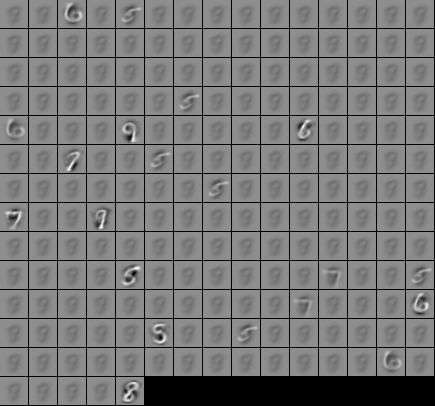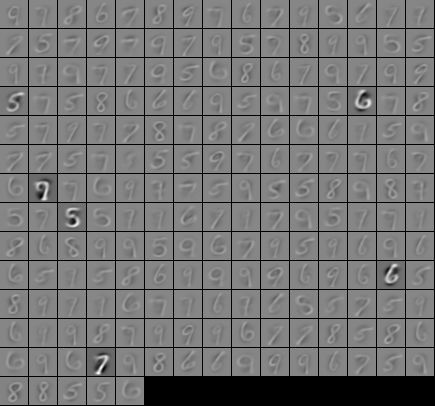## Sunday, 13 September 2015

### Deep learning 03--Self-Taught Learning and Unsupervised Feature Learning with Shark

Shark is a fast, modular, feature-rich open-source C++ machine learning library. Is it? At least it is not fast at all without the support of Atlas. Without Atlas, I took almost 2.5 hours to train about 29000 samples of MNIST on windows 8 64bits using sparse autoencoder with 200 iterations. So why not I compile and link to Atlas? The bad news is, Atlas is difficult to build under windows and not well optimized for windows 64bits.My conclusion is, if you really want to use shark to do some serious training, change your OS.I will use ubuntu or other OS if I want to continue to use Shark, without Atlas the performance is unable to accept.The other lesson I learn from Shark is, if you want your libraries/apps portable, never ever develop your libraries/apps on top of those hard to compile libraries.

Even the speed of Shark is quite slow without Atlas, it is still a modular, feature-rich machine learning library, I am quite impress about how good it split and combine different piece of concepts together. With the good design architecture of Shark, you can try out different results of algorithms with a few lines of codes, they are easy to read and elegant.

I use Shark to solve one of the exercise of UFLDL , a pleasant experience except of the speed(without Atlas). I use 6 different Autoencoder to train the features than feed into random forest to classify the hand written digits from 5~9. The beauty of Shark is, I only need to do some changes to try out these 5(at first it is 8, but three of them are too damn slow or buggy) algorithms.

First, you need to define the type of the autoencoder.

```using Autoencoder1 =
shark::Autoencoder<shark::LogisticNeuron, shark::LogisticNeuron>;

using Autoencoder2 =
shark::TiedAutoencoder<shark::LogisticNeuron, shark::LogisticNeuron>;

using Autoencoder3 =
shark::Autoencoder<
shark::DropoutNeuron<shark::LogisticNeuron>,
shark::LogisticNeuron
>;

using Autoencoder4 =
shark::TiedAutoencoder<
shark::DropoutNeuron<shark::LogisticNeuron>,
shark::LogisticNeuron
>;
```

2 :  the sparse autoencoder and autoencoder use different cost functions, so I need to change the cost functions from ErrorFunction to SparseAutoEncoderError.

3 :  sparse autoencoder cannot get good results with IRpropPlusFull, I need to use LBFGS to replace it.

4 : The last thing is, the initial bias value of sparse autoencoder should be zero.

Combine 2,3,4,  I decided to put them into different functions.

```template<typename Optimizer, typename Error, typename Model>
std::string optimize_params(std::string const &encoder_name,
size_t iterate,
Optimizer *optimizer,
Error *error,
Model *model)
{
using namespace shark;

Timer timer;
std::ostringstream str;
for (size_t i = 0; i != iterate; ++i) {
optimizer->step(*error);
str<<i<<" Error: "<<optimizer->solution().value <<"\n";
}
str<<"Elapsed time: " <<timer.stop()<<"\n";
str<<"Function evaluations: "<<error->evaluationCounter()<<"\n";

exportFiltersToPGMGrid(encoder_name, model->encoderMatrix(), 28, 28);
std::ofstream out(encoder_name);
boost::archive::polymorphic_text_oarchive oa(out);
model->write(oa);

return str.str();
}

template<typename Model>
std::string train_autoencoder(std::vector<shark::RealVector> const &unlabel_data,
std::string const &encoder_name,
Model *model)
{
using namespace shark;

model->setStructure(unlabel_data.size(), 200);
initRandomUniform(*model, -0.1*std::sqrt(1.0/unlabel_data.size()),
0.1*std::sqrt(1.0/unlabel_data.size()));

SquaredLoss<RealVector> loss;
UnlabeledData<RealVector> const Samples = createDataFromRange(unlabel_data);
RegressionDataset data(Samples, Samples);

ErrorFunction error(data, model, &loss);
const double lambda = 0.01; // Weight decay paramater
TwoNormRegularizer regularizer(error.numberOfVariables());
error.setRegularizer(lambda, &regularizer);

//output some info of model, like number of params, input size etc
output_model_state(*model);

IRpropPlusFull optimizer;
optimizer.init(error);
return optimize_params(encoder_name, 200, &optimizer, &error, model);
}

template<typename Model>
std::string train_sparse_autoencoder(std::vector<shark::RealVector> const &unlabel_data,
std::string const &encoder_name,
Model *model)
{
using namespace shark;

model->setStructure(unlabel_data.size(), 200);
if(std::is_same<Model, Autoencoder2>::value ||
std::is_same<Model, Autoencoder4>::value){
initRandomUniform(*model, -0.1*std::sqrt(1.0/unlabel_data.size()),
0.1*std::sqrt(1.0/unlabel_data.size()));
}else{
initialize_ffnet(model);
}

SquaredLoss<RealVector> loss;
UnlabeledData<RealVector> const Samples = createDataFromRange(unlabel_data);
RegressionDataset data(Samples, Samples);

const double Rho = 0.01; // Sparsity parameter
const double Beta = 6.0; // Regularization parameter
SparseAutoencoderError error(data, model, &loss, Rho, Beta);
const double lambda = 0.01; // Weight decay paramater
TwoNormRegularizer regularizer(error.numberOfVariables());
error.setRegularizer(lambda, &regularizer);

//output some info of model, like number of params, input size etc
output_model_state(*model);

LBFGS optimizer;
optimizer.lineSearch().lineSearchType() = LineSearch::WolfeCubic;
optimizer.init(error);
return optimize_params(encoder_name, 400, &optimizer, &error, model);
}

```

After I have the train function, the training process become quite easy to write.

```void autoencoder_prediction(std::vector<shark::RealVector> const &train_data,
std::vector<unsigned int> const &train_label)
{
{
Autoencoder1 model;
train_autoencoder(train_data, "ls_ls.txt", &model);
prediction("ls_ls.txt", "ls_ls_rtree.txt", train_data,
train_label, &model);
}

{
Autoencoder2 model;
train_autoencoder(train_data, "tied_ls_ls.txt", &model);
prediction("tied_ls_ls.txt", "tied_ls_ls_rtree.txt", train_data,
train_label, &model);
}

//Autoencoder3 has bug, the prediction will stuck and cannot complete
//Do not know it is cause by Shark3.0 beta or my fault
/*
{
Autoencoder3 model;
train_autoencoder(train_data, "dropls_ls.txt", &model);
prediction("dropls_ls.txt", "dropls_ls_rtree.txt", train_data,
train_label, &model);
});//*/

{
Autoencoder4 model;
train_autoencoder(train_data, "tied_dropls_ls.txt", &model);
prediction("tied_dropls_ls.txt", "tied_dropls_ls_rtree.txt", train_data,
train_label, &model);
}
}
```

All of the algorithms use same function to train and predict, I only need to change the type of the autocoder and file names(the files will save the result of training).

The part of prediction is almost same as the example of Shark, the different part is this example train and test on the data of MNIST. The codes are locate at github.

Results of autoencoder

Autoencoder1: iterate 200 times
Random Forest on training set accuracy: 1
Random Forest on test set accuracy: 0.978194

Autoencoder2: iterate 200 times
Random Forest on training set accuracy: 1
Random Forest on test set accuracy: 0.9784
Autoencoder4:iterate 200 times
Random Forest on training set accuracy: 1
Random Forest on test set accuracy: 0.918741

Results of sparse autoencoder
Autoencoder1: iterate 400 times
Random Forest on training set accuracy: 1
Random Forest on test set accuracy: 0.920798

Autoencoder2: iterate 200 times
Random Forest on training set accuracy: 1
Random Forest on test set accuracy: 0.95721

Visualization of the train results of autoencoder

Visualization of the train results of sparse autoencoderAutoencoder1Autoencoder2

Okay, Shark is slow on windows, it is almost impossible to expect Shark can manage real time image recognition task, is it possible to develop real time image recognition application with deep learning algo without rewrite?Maybe caffe can save my ass, according to stackoverflow, CNN perform better than deep belief network if you are dealing with computer vision tasks. What if I really need a fast and stable autoencoder on windows?Maybe mlpack could be an option(no guarantee it could be build on windows). If I only want to do some research, using R or python maybe is a better solution, since they are easier to install and provide good performance, but I need to use c++ to create real world products, that is why a decent machine learning library is crucial for me.